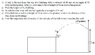# Projectile Problem: Solve for Height of Building & More

• Lord Dark
In summary, the conversation discusses a problem involving a projectile being thrown from the top of a building and its height and velocity at various points in time. The conversation includes attempts at solving for the height of the building, a second time when the ball is at a certain height, and the distance of the building from a wall when the ball hits it. The final equation used to solve for the height of the building is 84+h(b)=40sin(53)*2-0.5*9.8*4, and the results for the second time are t1=1.9s and t2=4.5s. The conversation also discusses finding the magnitude and direction of the velocity of the ball when it reaches the wall

## Homework Statement

hi guys ,, got this problem about projectile ,,
A ball is thrown from the top of a building with a velocity of 40 m/s at an angle of 53
with the horizontal. After 2 s, it is seen to be at height of 84 m above the ground.
a) Find the height of the building
b) At which other time will the ball again be at a height of 84 m?
c) If the ball hits a wall at a height of 52 m above the ground, what is the distance of the
wall from the building?
d) Find the magnitude and direction of the velocity of the ball when it reaches the wall

## The Attempt at a Solution

i tried to get the height of the building by 84+h(b)=40sin(53)*2-9.8*4 ,, and I got h(b)=59.31m ,, anyway ,, the way is right ?? ,, and about (b) i tried to get the second time ,, but i get it negative (59.31+84=40sin(53)x-9.8*x^2 = 9.8x^2-40sin(53)x-143.31 and i get x=5.7 , x=-2.5 and i think it's wrong because none of them equal 2 ,, and one of them should equal 2 ,, any ideas what did I do wrong here ?

#### Attachments

•Q3.JPG
35.6 KB · Views: 349
Lord Dark said:
i tried to get the height of the building by 84+h(b)=40sin(53)*2-9.8*4 ,, and I got h(b)=59.31m ,, anyway ,, the way is right ??
Your expression for vertical position is not quite right. What's the general formula?

delta(y)=Voyt-0.5*g*t^2 ,, (I forgot half) thanks :D

now i get the height 72m ( which i think it's too high ) and i still can't get the second time,

Lord Dark said:
now i get the height 72m ( which i think it's too high ) and i still can't get the second time,
Show the final equation that you solved, with the numbers that you used.

123.71=40sin(53)t-0.5*9.8*t^2 ,, now i don't even get an answer (they are imaginary numbers)

Lord Dark said:
123.71=40sin(53)t-0.5*9.8*t^2 ,, now i don't even get an answer (they are imaginary numbers)
Show me the equation that you used to solve for the height of the building.

123.71=40sin(53)t-0.5*9.8*t^2 ,, now i don't even get an answer (they are imaginary numbers)

Lord Dark said:
123.71=40sin(53)t-0.5*9.8*t^2 ,, now i don't even get an answer (they are imaginary numbers)
Show me the equation that you used to solve for the height of the building. (There should be some variable representing the height in there somewhere.)

84+h(b)=40sin(53)*2-0.5*9.8*4 (Y-Yo=Voy*t-0.5*g*t^2)

Lord Dark said:
84+h(b)=40sin(53)*2-0.5*9.8*4 (Y-Yo=Voy*t-0.5*g*t^2)
Compare the first equation to the one in parentheses. (Careful with signs.)

... Did it ,, (84-39.71)=40sin(53)t-0.5*9.8*t^2 and i get t1=1.9 = 2s t2=4.5 s ,, about (c) = first I got t=6.1s then (X=Vox*t) =147.1m
(d) Vx=24 m/s (never change) Vy=-27.8 then get lVl and get the angle which is -49

thanks :D